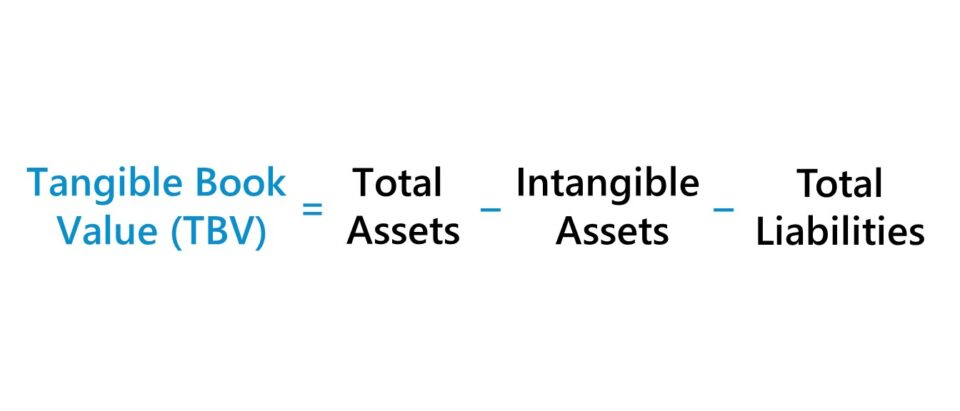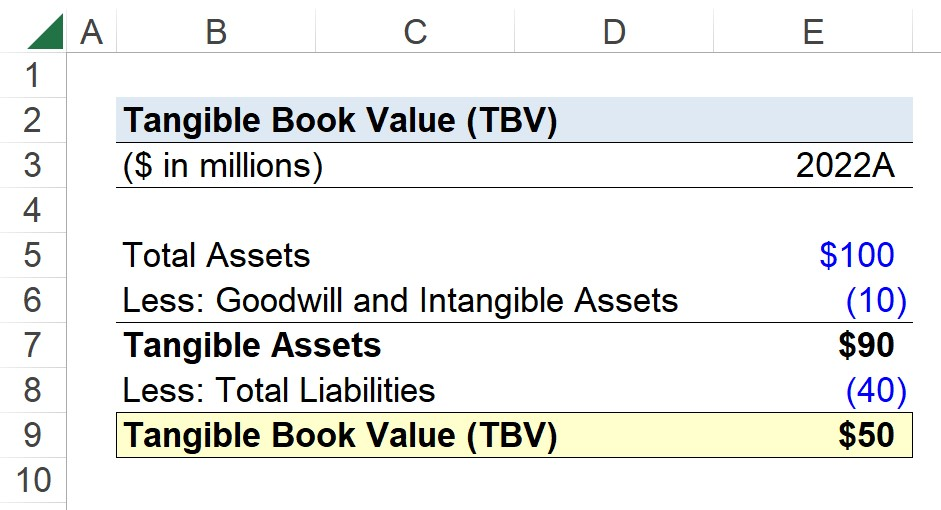Welcome to Wall Street Prep! Use code at checkout for 15% off.# Tangible Book Value (TBV)

Guide to Understanding Tangible Book Value (TBV)## How to Calculate Tangible Book Value (TBV)?

The tangible book value (TBV) measures how much a company’s tangible assets are worth, excluding its intangible assets.

• Tangible Assets: Physical Assets (e.g. Cash, Inventory, PP&E)
• Intangible Assets: Non-Physical Assets (e.g. Intellectual Property, Copyright, Patents, Trademarks, Goodwill)

Conceptually, the tangible book value (TBV) is the residual net value of a company that belongs to common shareholders post-liquidation, i.e. the remaining value once all outstanding liabilities like debt are repaid.

Intangible assets are excluded from the tangible book value calculation because such assets cannot be liquidated and sold off.

For instance, an intangible asset like goodwill is recognized on a company’s balance sheet for accounting purposes to capture the excess purchase price paid over the fair market value (FMV) of an asset.

Since only tangible assets are included in the calculation, the TBV is a closer estimate of the value of a company if it were to hypothetically file for bankruptcy and undergo a liquidation.

However, TBV is still an approximation rather than the actual value of a liquidated company, since certain intangible assets can still in fact be sold and the liquidation value of tangible assets is rarely perfectly equal to the full value recorded on the books.

## Tangible Book Value Formula (TBV)

The formula to calculate the tangible book value (TBV) is as follows.

Tangible Book Value (TBV) = (Total Assets  Intangible Assets)  Total Liabilities

The first part of the equation – i.e. total assets minus intangible assets – results in the value of a company’s tangible assets.

The value of all outstanding liabilities is then deducted from the value of the company’s tangible assets because claims such as debt and accounts payable are of higher priority and must be fulfilled before any proceeds can be distributed to common equity shareholders.

## Tangible Book Value Calculator (TBV)

We’ll now move on to a modeling exercise, which you can access by filling out the form below.Submitting...

## Tangible Book Value Calculation Example (TBV)

Suppose you’re tasked with calculating the tangible book value (TBV) of a company with the following balance sheet data.

• Total Assets = \$100 million
• Goodwill and Intangible Assets = \$10 million
• Total Liabilities = \$40 million

To start, we’ll deduct goodwill and intangible assets from total assets, which results in \$90 million of tangible assets.

• Tangible Assets = \$100 million – \$10 million = \$90 million

From there, the next step is to net the company’s tangible assets against its total liabilities.

• Tangible Book Value (TBV) = \$90 million – \$40 million = \$50 million

In conclusion, the tangible book value (TBV) of our hypothetical company is \$50 million.Step-by-Step Online Course

### Everything You Need To Master Financial Modeling

Enroll in The Premium Package: Learn Financial Statement Modeling, DCF, M&A, LBO and Comps. The same training program used at top investment banks.